# RS Aggarwal Solutions For Class 7 Maths Exercise 2A Chapter 2 Fractions

The pdf of RS Aggarwal Solutions for the Exercise 2A of Class 7 Maths Chapter 2, Fractions are available here. To cover the entire syllabus in Maths, the RS Aggarwal is an essential material as it offers a wide range of questions that test the students’ understanding of concepts. Our expert personnel have solved the problems step by step with neat explanations. Students who aim to score good marks in Maths, then refer RS Aggarwal Solutions for Class 7.

## Download the PDF of RS Aggarwal Solutions For Class 7 Maths Chapter 2 Fractions – Exercise 2A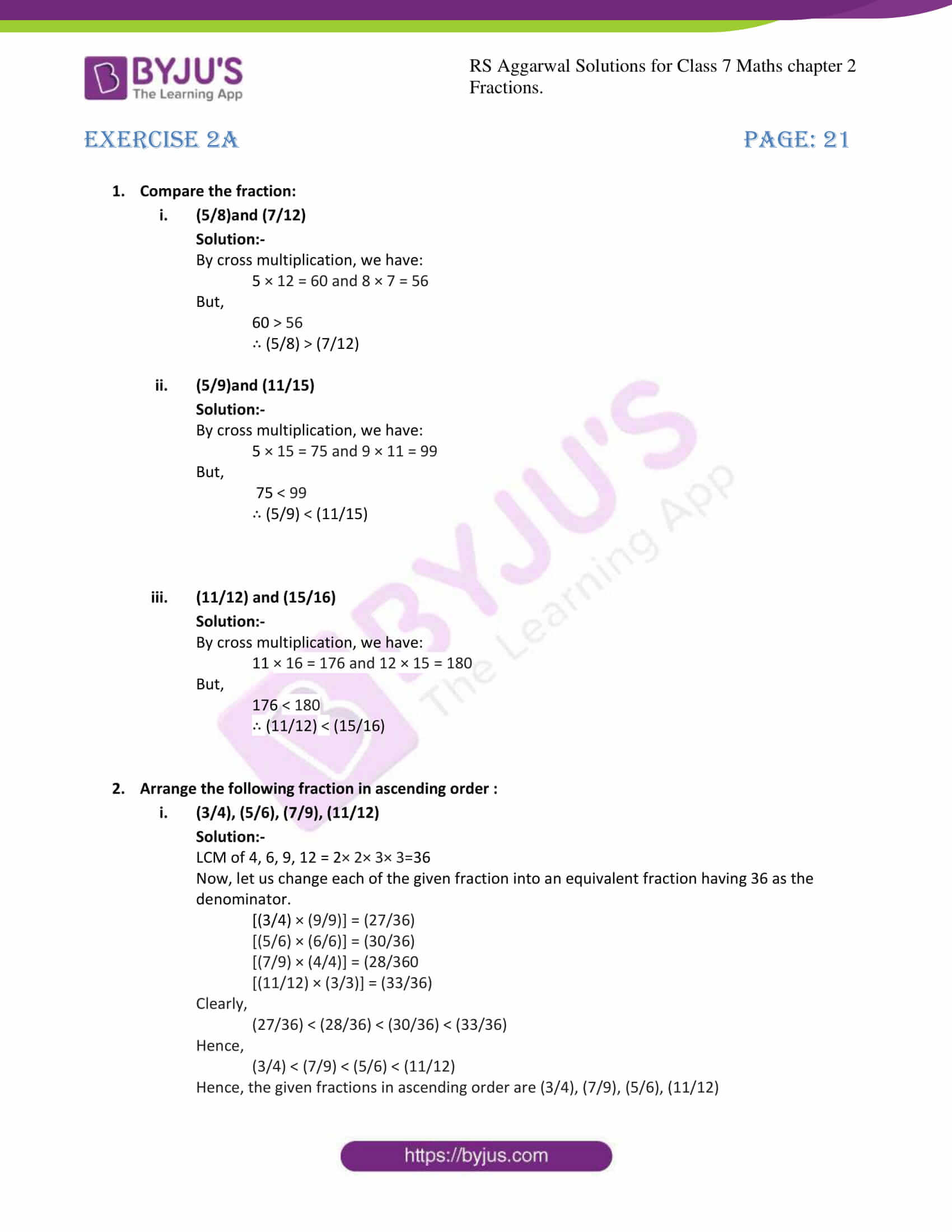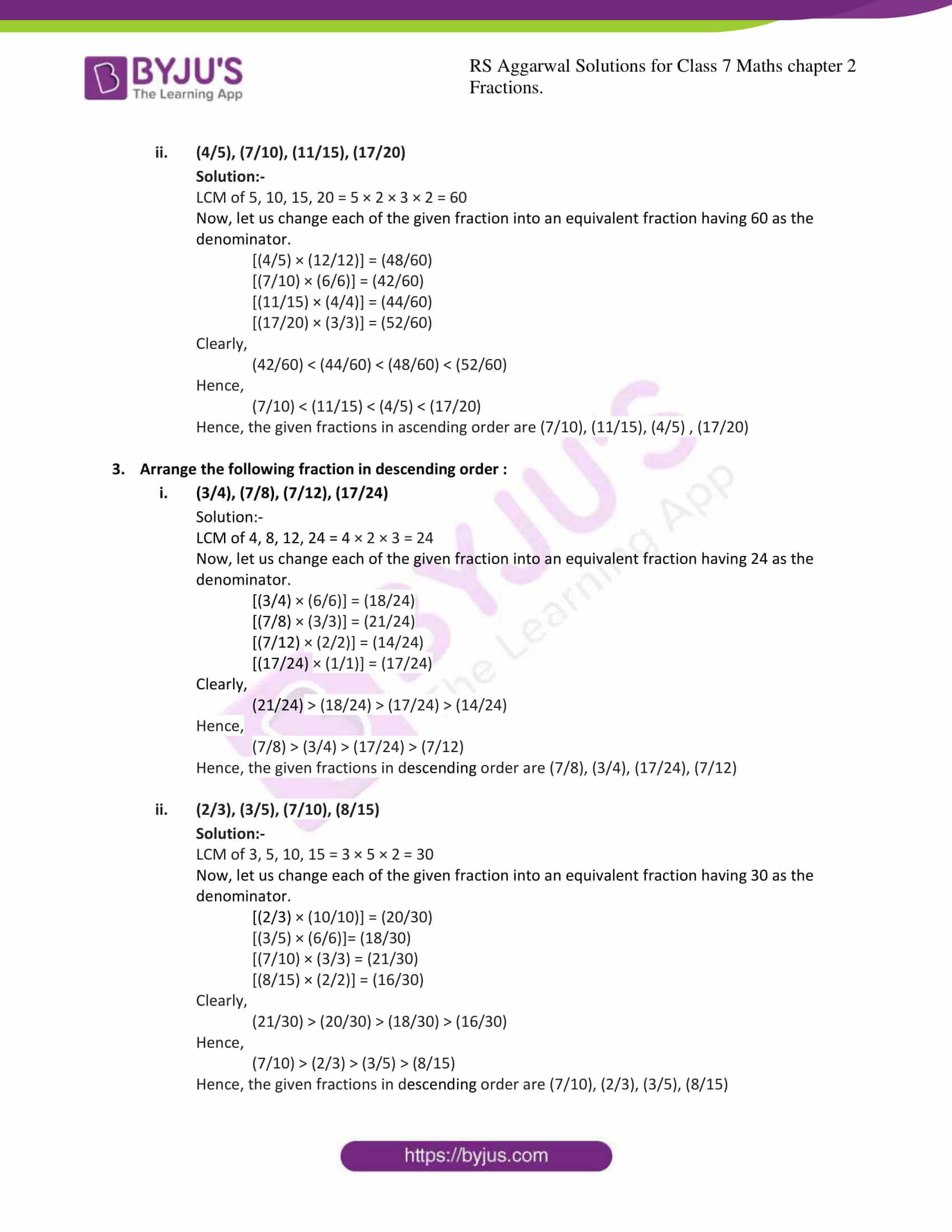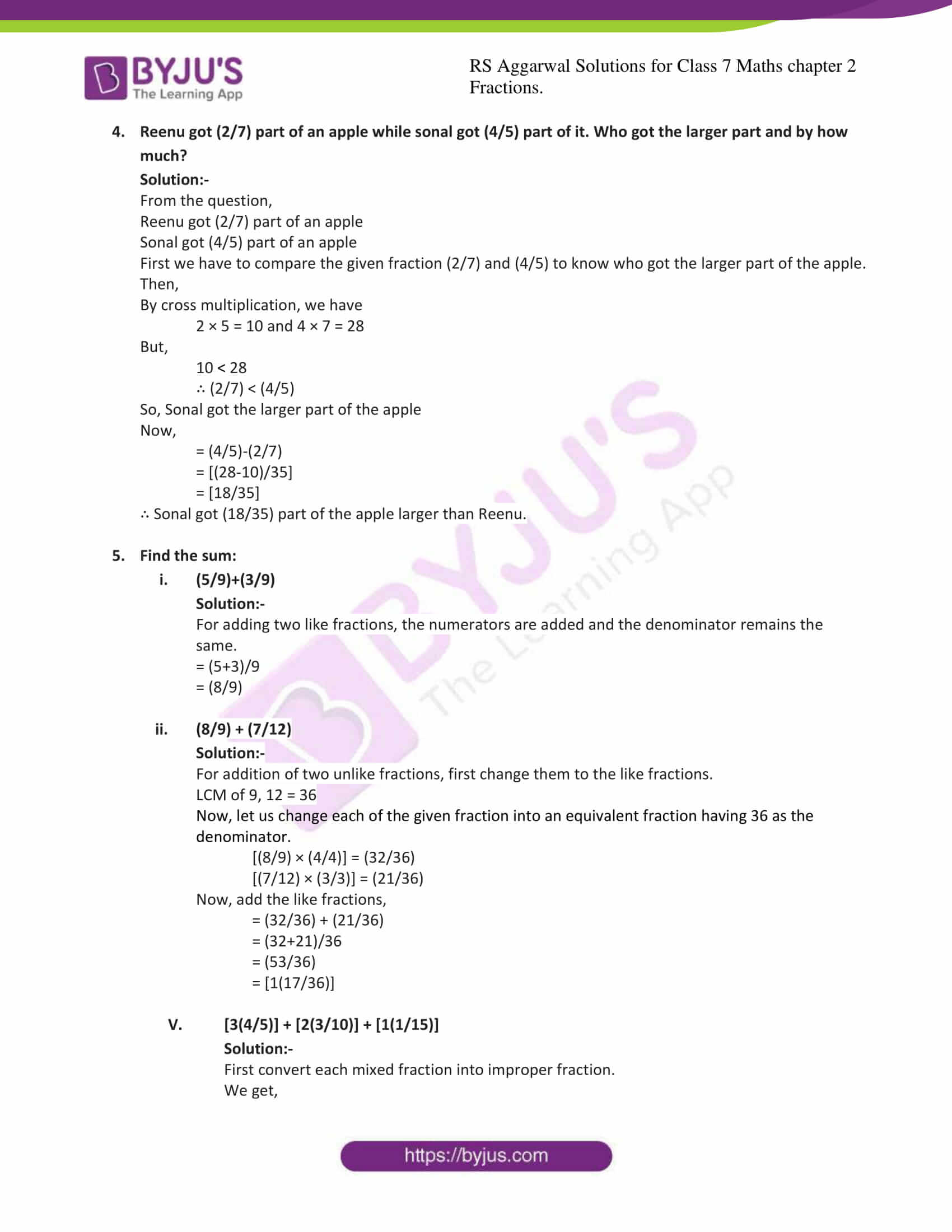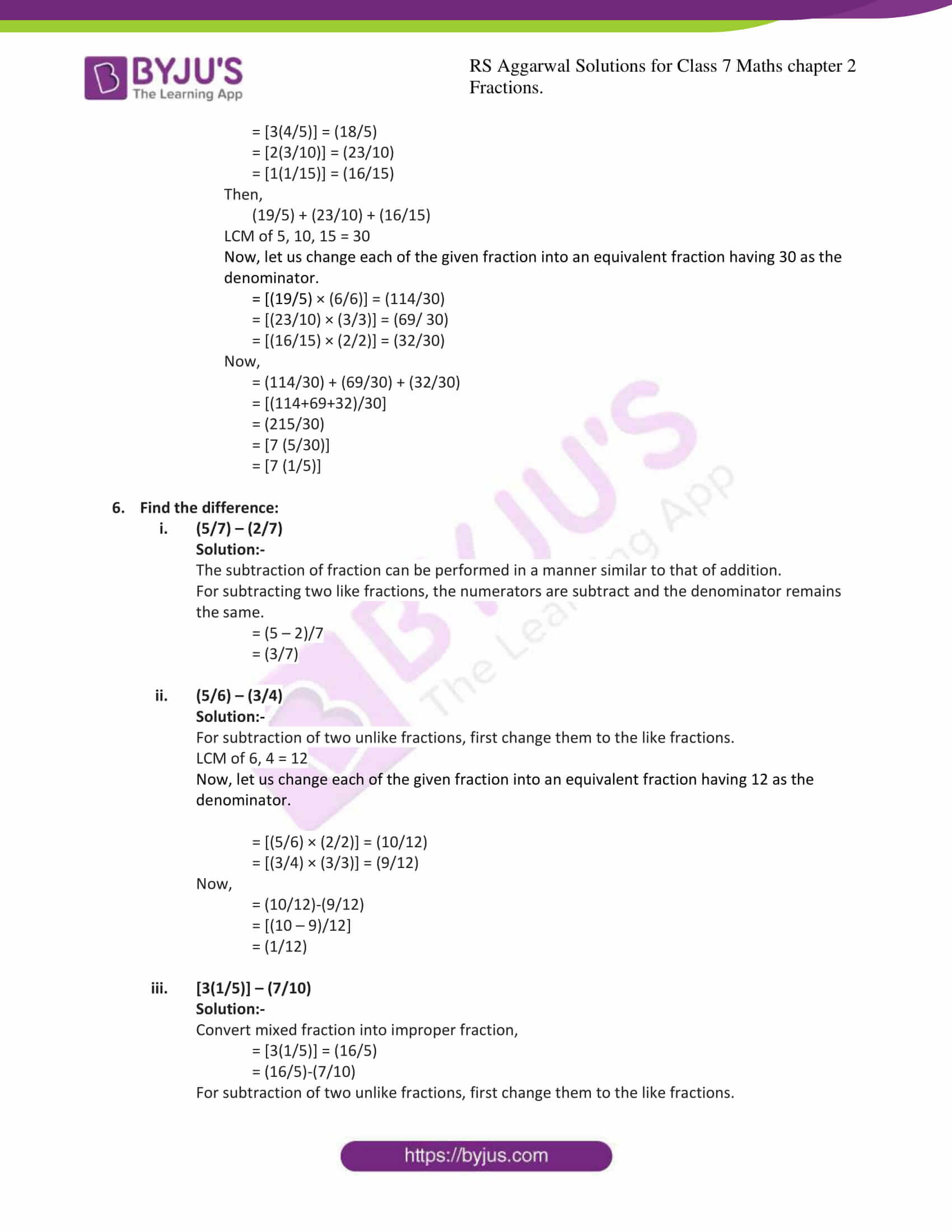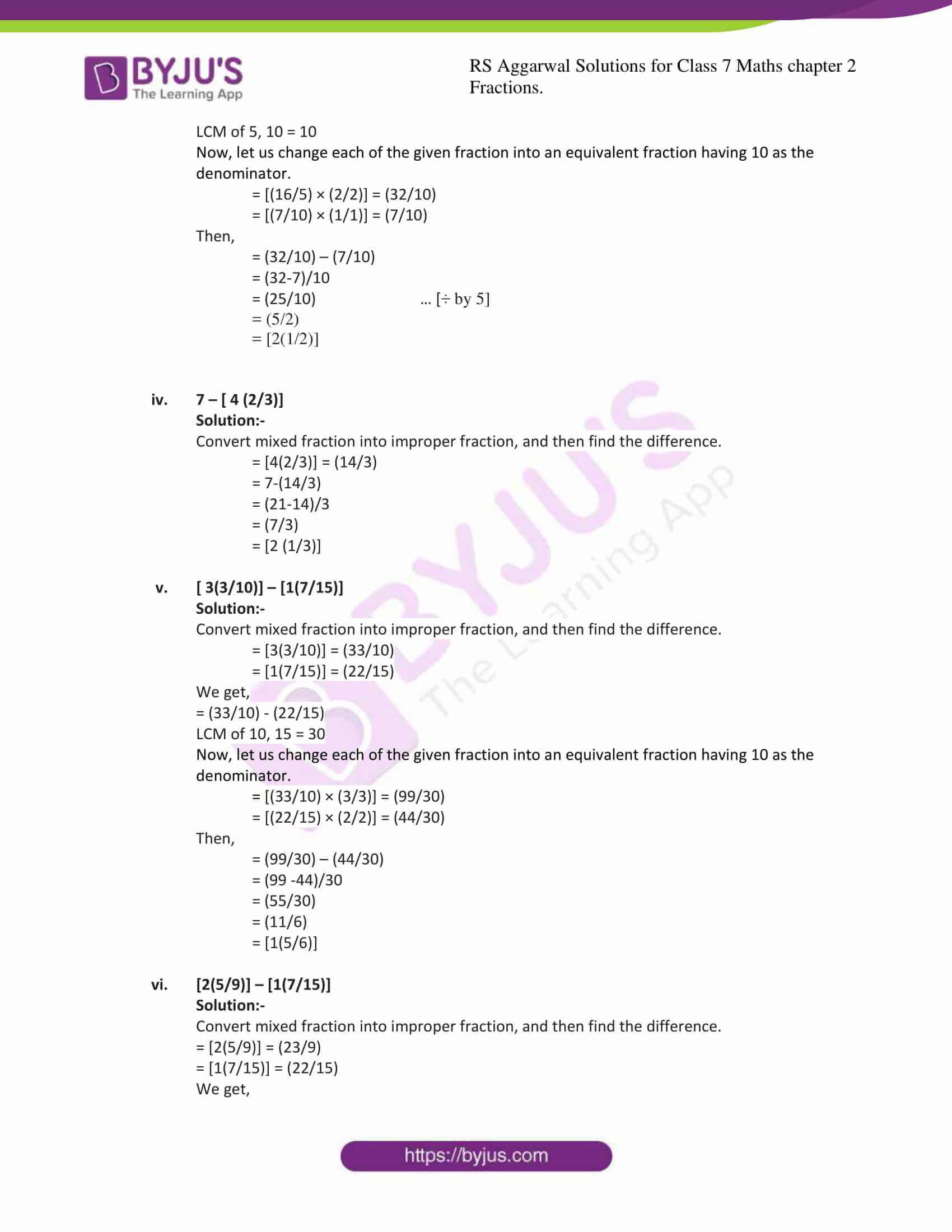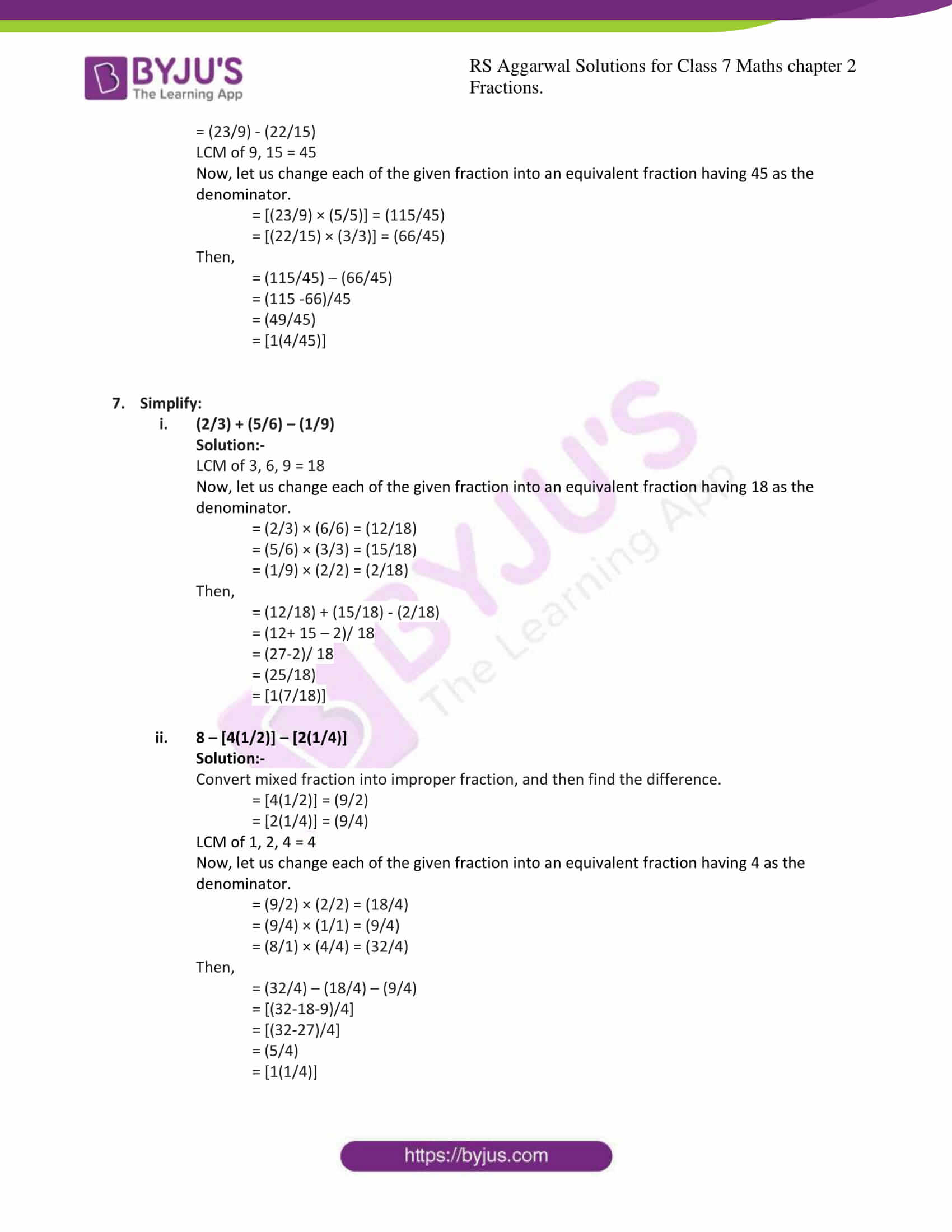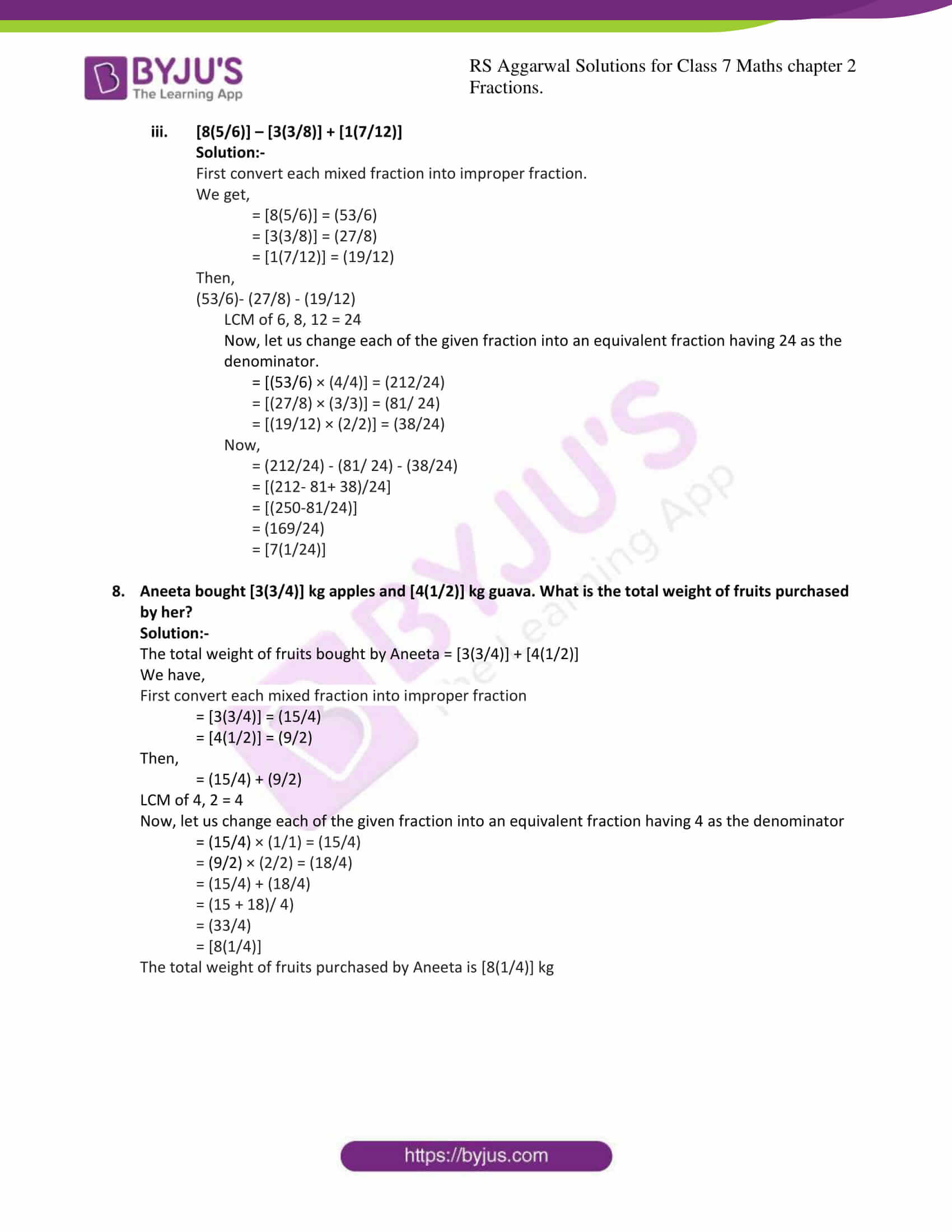### Access answers to Maths RS Aggarwal Solutions for Class 7 Chapter 2 – Fractions Exercise 2A

1. Compare the fraction:
(i) (5/8)and (7/12)

Solution:-

By cross multiplication, we have:

5 × 12 = 60 and 8 × 7 = 56

But,

60 > 56

∴ (5/8) > (7/12)

(ii) (5/9)and (11/15)

Solution:-

By cross multiplication, we have:

5 × 15 = 75 and 9 × 11 = 99

But,

75 < 99

∴ (5/9) < (11/15)

(iii) (11/12) and (15/16)

Solution:-

By cross multiplication, we have:

11 × 16 = 176 and 12 × 15 = 180

But,

176 < 180

∴ (11/12) < (15/16)

2. Arrange the following fraction in ascending order :
(i) (3/4), (5/6), (7/9), (11/12)

Solution:-

LCM of 4, 6, 9, 12 = 2× 2× 3× 3=36

Now, let us change each of the given fraction into an equivalent fraction having 36 as the denominator.

[(3/4) × (9/9)] = (27/36)

[(5/6) × (6/6)] = (30/36)

[(7/9) × (4/4)] = (28/360

[(11/12) × (3/3)] = (33/36)

Clearly,

(27/36) < (28/36) < (30/36) < (33/36)

Hence,

(3/4) < (7/9) < (5/6) < (11/12)

Hence, the given fractions in ascending order are (3/4), (7/9), (5/6), (11/12)

(ii) (4/5), (7/10), (11/15), (17/20)

Solution:-

LCM of 5, 10, 15, 20 = 5 × 2 × 3 × 2 = 60

Now, let us change each of the given fraction into an equivalent fraction having 60 as the denominator.

[(4/5) × (12/12)] = (48/60)

[(7/10) × (6/6)] = (42/60)

[(11/15) × (4/4)] = (44/60)

[(17/20) × (3/3)] = (52/60)

Clearly,

(42/60) < (44/60) < (48/60) < (52/60)

Hence,

(7/10) < (11/15) < (4/5) < (17/20)

Hence, the given fractions in ascending order are (7/10), (11/15), (4/5) , (17/20)

3. Arrange the following fraction in descending order :
(i) (3/4), (7/8), (7/12), (17/24)

Solution:-

LCM of 4, 8, 12, 24 = 4 × 2 × 3 = 24

Now, let us change each of the given fraction into an equivalent fraction having 24 as the denominator.

[(3/4) × (6/6)] = (18/24)

[(7/8) × (3/3)] = (21/24)

[(7/12) × (2/2)] = (14/24)

[(17/24) × (1/1)] = (17/24)

Clearly,

(21/24) > (18/24) > (17/24) > (14/24)

Hence,

(7/8) > (3/4) > (17/24) > (7/12)

Hence, the given fractions in descending order are (7/8), (3/4), (17/24), (7/12)

(ii) (2/3), (3/5), (7/10), (8/15)

Solution:-

LCM of 3, 5, 10, 15 = 3 × 5 × 2 = 30

Now, let us change each of the given fraction into an equivalent fraction having 30 as the denominator.

[(2/3) × (10/10)] = (20/30)

[(3/5) × (6/6)]= (18/30)

[(7/10) × (3/3) = (21/30)

[(8/15) × (2/2)] = (16/30)

Clearly,

(21/30) > (20/30) > (18/30) > (16/30)

Hence,

(7/10) > (2/3) > (3/5) > (8/15)

Hence, the given fractions in descending order are (7/10), (2/3), (3/5), (8/15)

4. Reenu got (2/7) part of an apple while sonal got (4/5) part of it. Who got the larger part and by how much?

Solution:-

From the question,

Reenu got (2/7) part of an apple

Sonal got (4/5) part of an apple

First we have to compare the given fraction (2/7) and (4/5) to know who got the larger part of the apple.

Then,

By cross multiplication, we have

2 × 5 = 10 and 4 × 7 = 28

But,

10 < 28

∴ (2/7) < (4/5)

So, Sonal got the larger part of the apple

Now,

= (4/5)-(2/7)

= [(28-10)/35]

= [18/35]

∴ Sonal got (18/35) part of the apple larger than Reenu.

5. Find the sum:
(i) (5/9)+(3/9)

Solution:-

For adding two like fractions, the numerators are added and the denominator remains the same.

= (5+3)/9

= (8/9)

(ii) (8/9) + (7/12)

Solution:-

For addition of two unlike fractions, first change them to the like fractions.

LCM of 9, 12 = 36

Now, let us change each of the given fraction into an equivalent fraction having 36 as the denominator.

[(8/9) × (4/4)] = (32/36)

[(7/12) × (3/3)] = (21/36)

= (32/36) + (21/36)

= (32+21)/36

= (53/36)

= [1(17/36)]

(iii) [3(4/5)] + [2(3/10)] + [1(1/15)]

Solution:-

First convert each mixed fraction into improper fraction.

We get,

= [3(4/5)] = (18/5)

= [2(3/10)] = (23/10)

= [1(1/15)] = (16/15)

Then,

(19/5) + (23/10) + (16/15)

LCM of 5, 10, 15 = 30

Now, let us change each of the given fraction into an equivalent fraction having 30 as the denominator.

= [(19/5) × (6/6)] = (114/30)

= [(23/10) × (3/3)] = (69/ 30)

= [(16/15) × (2/2)] = (32/30)

Now,

= (114/30) + (69/30) + (32/30)

= [(114+69+32)/30]

= (215/30)

= [7 (5/30)]

= [7 (1/5)]

6. Find the difference:
(i) (5/7) – (2/7)

Solution:-

The subtraction of fraction can be performed in a manner similar to that of addition.

For subtracting two like fractions, the numerators are subtract and the denominator remains the same.

= (5 – 2)/7

= (3/7)

(ii) (5/6) – (3/4)

Solution:-

For subtraction of two unlike fractions, first change them to the like fractions.

LCM of 6, 4 = 12

Now, let us change each of the given fraction into an equivalent fraction having 12 as the denominator.

= [(5/6) × (2/2)] = (10/12)

= [(3/4) × (3/3)] = (9/12)

Now,

= (10/12)-(9/12)

= [(10 – 9)/12]

= (1/12)

(iii) [3(1/5)] – (7/10)

Solution:-

Convert mixed fraction into improper fraction,

= [3(1/5)] = (16/5)

= (16/5)-(7/10)

For subtraction of two unlike fractions, first change them to the like fractions.

LCM of 5, 10 = 10

Now, let us change each of the given fraction into an equivalent fraction having 10 as the denominator.

= [(16/5) × (2/2)] = (32/10)

= [(7/10) × (1/1)] = (7/10)

Then,

= (32/10) – (7/10)

= (32-7)/10

= (25/10) … [÷ by 5]

= (5/2)

= [2(1/2)]

(iv) 7 – [ 4 (2/3)]

Solution:-

Convert mixed fraction into improper fraction, and then find the difference.

= [4(2/3)] = (14/3)

= 7-(14/3)

= (21-14)/3

= (7/3)

= [2 (1/3)]

(v) [ 3(3/10)] – [1(7/15)]

Solution:-

Convert mixed fraction into improper fraction, and then find the difference.

= [3(3/10)] = (33/10)

= [1(7/15)] = (22/15)

We get,

= (33/10) – (22/15)

LCM of 10, 15 = 30

Now, let us change each of the given fraction into an equivalent fraction having 10 as the denominator.

= [(33/10) × (3/3)] = (99/30)

= [(22/15) × (2/2)] = (44/30)

Then,

= (99/30) – (44/30)

= (99 -44)/30

= (55/30)

= (11/6)

= [1(5/6)]

(vi) [2(5/9)] – [1(7/15)]

Solution:-

Convert mixed fraction into improper fraction, and then find the difference.

= [2(5/9)] = (23/9)

= [1(7/15)] = (22/15)

We get,

= (23/9) – (22/15)

LCM of 9, 15 = 45

Now, let us change each of the given fraction into an equivalent fraction having 45 as the denominator.

= [(23/9) × (5/5)] = (115/45)

= [(22/15) × (3/3)] = (66/45)

Then,

= (115/45) – (66/45)

= (115 -66)/45

= (49/45)

= [1(4/45)]

7. Simplify:
(i) (2/3) + (5/6) – (1/9)

Solution:-

LCM of 3, 6, 9 = 18

Now, let us change each of the given fraction into an equivalent fraction having 18 as the denominator.

= (2/3) × (6/6) = (12/18)

= (5/6) × (3/3) = (15/18)

= (1/9) × (2/2) = (2/18)

Then,

= (12/18) + (15/18) – (2/18)

= (12+ 15 – 2)/ 18

= (27-2)/ 18

= (25/18)

= [1(7/18)]

(ii) 8 – [4(1/2)] – [2(1/4)]

Solution:-

Convert mixed fraction into improper fraction, and then find the difference.

= [4(1/2)] = (9/2)

= [2(1/4)] = (9/4)

LCM of 1, 2, 4 = 4

Now, let us change each of the given fraction into an equivalent fraction having 4 as the denominator.

= (9/2) × (2/2) = (18/4)

= (9/4) × (1/1) = (9/4)

= (8/1) × (4/4) = (32/4)

Then,

= (32/4) – (18/4) – (9/4)

= [(32-18-9)/4]

= [(32-27)/4]

= (5/4)

= [1(1/4)]

(iii) [8(5/6)] – [3(3/8)] + [1(7/12)]

Solution:-

First convert each mixed fraction into improper fraction.

We get,

= [8(5/6)] = (53/6)

= [3(3/8)] = (27/8)

= [1(7/12)] = (19/12)

Then,

(53/6)- (27/8) – (19/12)

LCM of 6, 8, 12 = 24

Now, let us change each of the given fraction into an equivalent fraction having 24 as the denominator.

= [(53/6) × (4/4)] = (212/24)

= [(27/8) × (3/3)] = (81/ 24)

= [(19/12) × (2/2)] = (38/24)

Now,

= (212/24) – (81/ 24) – (38/24)

= [(212- 81+ 38)/24]

= [(250-81/24)]

= (169/24)

= [7(1/24)]

8. Aneeta bought [3(3/4)] kg apples and [4(1/2)] kg guava. What is the total weight of fruits purchased by her?

Solution:-

The total weight of fruits bought by Aneeta = [3(3/4)] + [4(1/2)]

We have,

First convert each mixed fraction into improper fraction

= [3(3/4)] = (15/4)

= [4(1/2)] = (9/2)

Then,

= (15/4) + (9/2)

LCM of 4, 2 = 4

Now, let us change each of the given fraction into an equivalent fraction having 4 as the denominator

= (15/4) × (1/1) = (15/4)

= (9/2) × (2/2) = (18/4)

= (15/4) + (18/4)

= (15 + 18)/ 4)

= (33/4)

= [8(1/4)]

The total weight of fruits purchased by Aneeta is [8(1/4)] kg

### RS Aggarwal Solutions for Class 7 Maths Exercise 2A Chapter – 2 Fractions

RS Aggarwal Solutions For Class 7 Maths Chapter 2 Fractions Exercise 2A has problems which are based on method of changing unlike fractions to like fractions, comparing of fractions, addition of fractions and subtraction of fractions. Students are suggested to try solving the questions from RS Aggarwal Solutions book of Class 7 and then refer to these solutions to know the best way of approaching the different questions.